Next: 7.5 Probabilistic Context-Free Grammars Up: Chapter7Ambiguity Resoltion: Statistical Methods Previous: Box 7.1 Getting Reliable

# 7.4 Obtaining Lexical Probabilities

• $BBg5,LO$J(B corpus $B$,EE;R2=$5$l$k$H(B,$B$=$N>pJs$H(B parser $B$r7k$SIU$1$F@:(B $BEY$N$h$$(B parsing Br9T&3H,G-kH4|BT5lk(B • B9YFN(B courpus B,@57$$(B data $B$J$i$P(B viterbi algorithm $B$N7k2L$r(B $B$=$N$^$^(B parser $B$KEO$;$P$h$$(B • B7+7(B,B9YFN(B courpus B,@57$$(B data $B$O8B$i$J$$(B, Bhj:GE,J2rr(B B5aakI,MW@-,"k(B • BHJkH(B, viterbi algorithm BNh&J:GBg(B category BN3djEvFr(B B?@RHD@15aakh&J(B algorithm BGOJ/(B, context Br9MN87F(B B3FC18lKM=A[5lk(B category BN3NN(r9YF5aaF(B, parser BKEO7?(B B[&,$$$$NGOJ$$$+(B?
$BF~NO(B context $B$N>r7o2<$G$NG$0U$NIJ;l(B,$BG$0U$NC18l0LCV$N=P8=3NN((B, P(wt/Li|Input) • context-independ $B$JC18l$N=P8=3NN((B Figure 7.13
• context $B$r9MN8$7$F(B The flies like flowers'' $B$NCf$G(B, The flies'' $B$N(B flies $B$,L>;l$G$"$k3NN($r5a$a$k(B.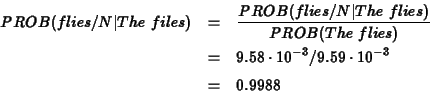• $BJ,;R(B
The/? flies/N $B$H$J$k9M$($&$k$9$Y$F$N3NN($NOB(B
The/ART flies/N (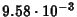)
The/N flies/N (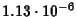)
The/P flies/N (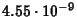)
• $BJ,Jl(B The/? flies/? $B$H$J$k9M$($&$k$9$Y$F3NN($NOB(B
The/? flies/V $B$H$&CM$b9MN8(B (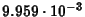)
$B$3$N(B context $B$G(B flies $B$,(B N $B$K$J$k3NN($O(B 0.9998
$B$3$N$h$&$J(B context $B$r9MN8$7$?3NN(CM$r8zN($h$/5a$a$k$K$O(B? viterbi algorithm $B$K;w$?forward probability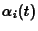•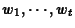$B$NC18lNs$,=P8=$7(B,$B$+$D(Bt $BHVL\$NC18l$,(B category i $B$KB0$9$k3NN((B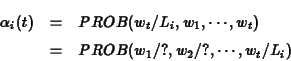• $B4pK\E*$K(B vitebi algorithm $B$HF1$8(B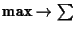$B$KJQ99(B (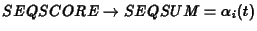) $B2DG=$JAH9g$o$;$N3NN($NOB(B • SEQSUM$B$NCM$G@55,2=(BFigure 7.14 $B;2>H(B • Figure 7.15 viterbi algorithm $B$G(B$B$KJQ99$7(B $B$?>l9g$N(B score (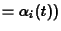• Figure 7.16$B$+$i5a$a$?3NN(CM(B
($BNc(B)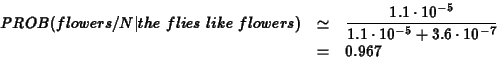• backward probability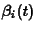•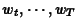$B$NC18lNs$,=P8=$7(B,$B$+$D(Bt $BHVL\$NC18l$,(B category i $B$KB0$9$k3NN((B • forward probability $B$HF1$8(B, $B5a$a$k8~$-$,5U(B, $BJ8Kv$+$i;O$^$k(B
• lexical probabilites for word wt
• forward brobability, backword probability $B$rAH$_9g$o$;$k(B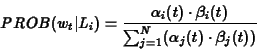1999-08-03